Next: 3.3 The Rotating Reference Up: 3.2 Measuring the Internal Previous: 3.2.4 Four-Counter Geometry and

## 3.2.5 The Fourier Transform

The Fourier transform of the complex muon polarization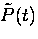gives a good approximation of the actual internal field distribution. The Fourier transform is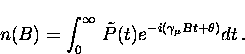(29)
Due to the finite counting rates, the Fourier transform contains statistical noise. The noisy or distorted portions of the asymmetry spectrum can be eliminated from the Fourier transform through apodization''. Apodization is achieved by multiplying the asymmetry spectrum by a weighting function which varies between one and zero . For example, the Fourier transform can be apodized with a Gaussian function so that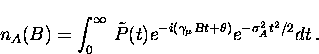(30)
Unfortunately, this apodization procedure also broadens the Fourier transform. The apodization parameter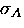is chosen to provide a compromise between the statistical noise in the spectrum and the additional broadening of the spectrum which such a procedure introduces. The Fourier transform will also appear broader than the actual field distribution in the sample because the muon spin precession signal is measured over a finite time interval. Because of the finite number of recorded events, the integral in Eq. (3.34) is replaced with a sum. Examples of the Fourier transform of the muon precession signal in the vortex state will be presented in the next chapter. Since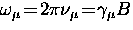, the Fourier transform can be presented as a function of either magnetic field B or the muon precession frequency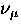.Next: 3.3 The Rotating Reference Up: 3.2 Measuring the Internal Previous: 3.2.4 Four-Counter Geometry and